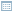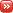• ## Naive Bayes in Layman's Terms

Posted by Konstantin 22.03.2015

This is a repost of my quora answer to the question: In layman's terms, how does Naive Bayes work?

Suppose that you are a working as a security guard at the airport. Your task is to look at people who pass the security line and pick some of them as being worthy of a more detailed screening. Now, of course, telling whether a person is a potential criminal or not by just looking at him/her is hard, if at all possible, but you need to do something. You have been put there for some reason, after all.

One of the simplest ways to approach the problem, mentally, is the following. You assign a "risk value" for each person. At the beginning (when you don't have any information about the person at all) you set this value to zero.

Now you start studying various features of the person in front of you: is it a male or a female? Is it a kid? Is he behaving nervously? Is he carrying a big bag? Is he alone? Did the metal detector beep? Is he a foreigner? etc. For each of those features you know (subconsciously due to your presuppositions, or from actual statistics) the average increase or decrease in risk of the person being a criminal that it entails. For example, if you know that the proportion of males among criminals is the same as the proportion of males among non-criminals, observing that a person is male will not affect his risk value at all. If, however, there are more males among criminals (suppose the percentage is, say, 70%) than among decent people (where the proportion is around 50%), observing that a person in front of you is a male will increase the "risk level" by some amount (the value is log(70%/50%) ~ 0.3, to be precise). Then you see that a person is nervous. OK, you think, 90% of criminals are nervous, but only 50% of normal people are. This means that nervousness should entail a further risk increase (of log(0.9/0.5) ~ 0.6, to be technical again, so by now you have counted a total risk value of 0.9). Then you notice it is a kid. Wow, there is only 1% of kids among criminals, but around 10% among normal people. Therefore, the risk value change due to this observation will be negative (log(0.01/0.10) ~ -2.3, so your totals are around -1.4 by now).

You can continue this as long as you want, including more and more features, each of which will modify your total risk value by either increasing it (if you know this particular feature is more representative of a criminal) or decreasing (if the features is more representative of a decent person). When you are done collecting the features, all is left for you is to compare the result with some threshold level. Say, if the total risk value exceeds 10, you declare the person in front of you to be potentially dangerous and take it into a detailed screening.

The benefit of such an approach is that it is rather intuitive and simple to compute. The drawback is that it does not take the cross-play of features into account. It may very well be the case that while the feature "the person is a kid" on its own greatly reduces the risk value, and the feature "has a moustache" on its own has close to no effect, a combination of the two ("a kid with a moustache") would actually have to increase the risk by a lot. This would not happen when you simply add the separate feature contributions, as described above.

Posted by Konstantin @ 4:27 pm

1. CODeRUS on 31.03.2016 at 01:15 (Reply)

Great explanation, thank you! But having found the risk value how do we go back to probabilities ? If I understand correctly, to do that, we can also calculate the opposite of risk, by taking e.g. log(50%/70%) instead of log(70%/50%), and then normalize the two values so that their sum equals 1. Is that correct ?

1. Konstantin on 31.03.2016 at 01:45 (Reply)

No, to get the posterior probability P(C|x) of your target class from the obtained "risk value" $r$ you need to use the sigmoid function:

$P(C|x) = \frac{1}{1+\exp(-r)}.$

In this case, though, to be completely formally correct, you need to make sure your final risk value also includes your "threshold" value (which corresponds to the prior $log \frac{P(C)}{P(\not C)}$). Alternatively, just start the reasoning with some initial, possibly nonzero, "prior" risk value, and use a zero threshold to decide).

To see why this is the case, observe that $r = \log \frac{P(C|x)}{P(\not C|x)}$ and substitute it in the sigmoid equation.

1. CODeRUS on 31.03.2016 at 03:06 (Reply)

Thank you! In this particular example, if I know that historically 0.01% of the passengers have been criminals, I can take log(10^(-4)) ~ -9 as the initial risk value and use it as a threshold to decide, right?

1. Konstantin on 31.03.2016 at 11:27 (Reply)

Now, your initial risk value in this case would be log(0.01/(1-0.01)) ~ -2.29, in which case your decision would simply compare the total with 0 (which would be the same as asking whether the posterior P(C|x) > 0.5).

Equivalently, you could have started with zero and used 2.29 as a decision threshold.

Note that the explanation in this post is not really meant for an in-depth technical understanding. If you want the latter, try deriving the algorithm mathematically, starting from the observation that

$\frac{P(C|x)}{P(\not C|x)} = \frac{P(x|C)P(C)}{P(x|\not C)P(\not C)}$

then using the conditional independence assumption

$P(x|C) = P(x_1|C)P(x_2|C)\dots$

and taking the logarithm.

Please note: Comment moderation is enabled and may delay your comment. There is no need to resubmit your comment.

### Calendar

March 2015
M T W T F S S
« Jan   Apr »
1
2345678
9101112131415
16171819202122
23242526272829
3031